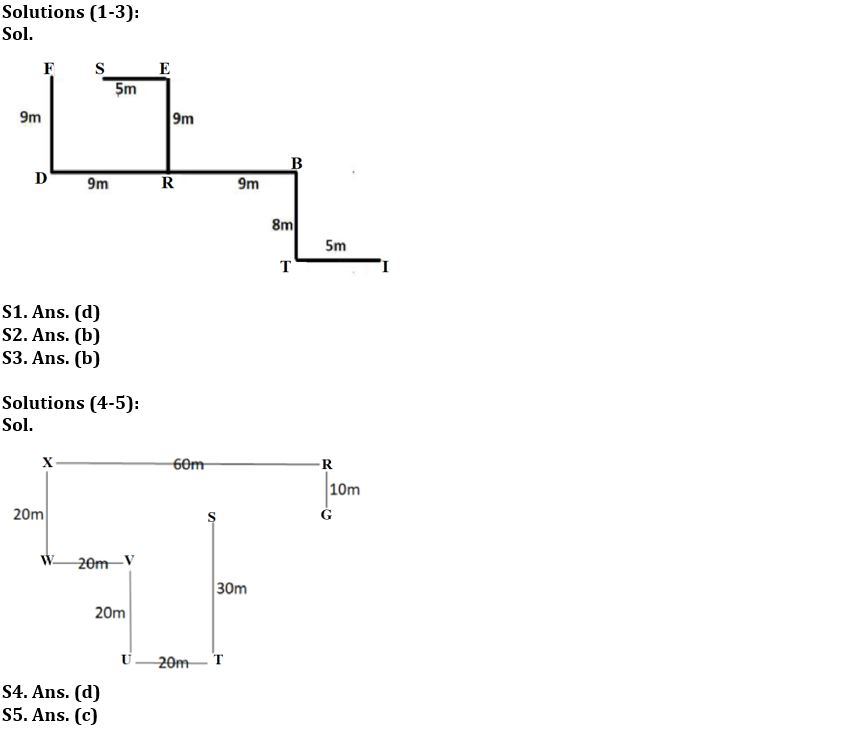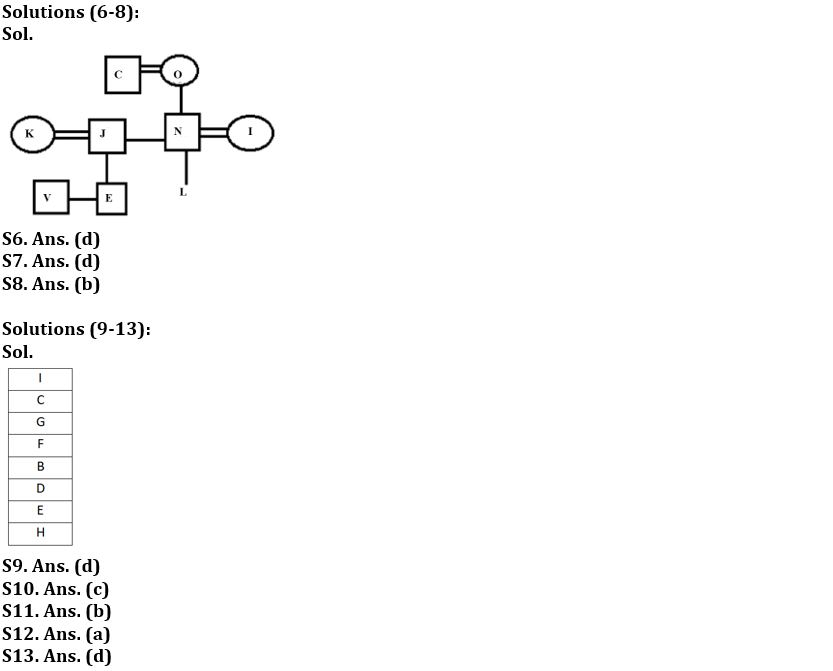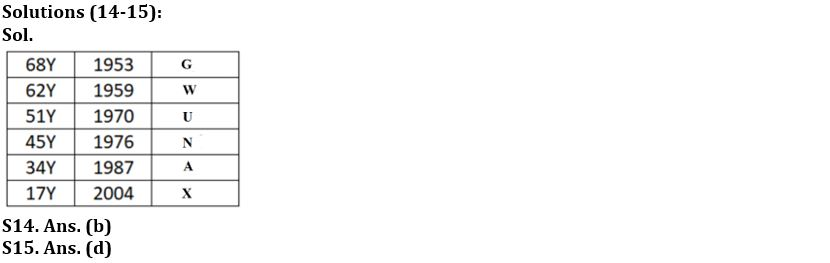Latest Banking jobs   »

# Reasoning Ability Quiz For FCI Phase I 2023- 4th January

Direction (1-3): Read the following information carefully and answer the questions which follow.
Point R is to the south of Point E. Point R is 9m to the west of Point B. Point F is 9m to the north of Point D. Point T is 8m to the south of Point B. Point F and E lie in the same straight line. Point R is the midpoint between Point B and D. Point I is 5m to the east of Point T.

Q1. What is the total distance between point F and point T?
(a) 42m
(b) 39m
(c) 44m
(d) 35m
(e) None of these

Q2. If point S is 5m west of point E, then what is the direction of point R with respect to point S?
(a) South
(b) South-east
(c) North-east
(d) North-west
(e) None of these

Q3. If point S is 5m west of point E, then which of the following points are to the north-west of Point B?
(a) Point S and Point I
(b) Point S, Point E and Point F
(c) Point E, Point S and Point T
(d) Point D and Point R
(e) None of these

Direction (4-5): Study the data carefully and answer the questions accordingly.
Point T is 30m south of point S. Point R is 60m east of point X. Point T is 20m east of point U. Point U is 20m south of point V. Point W is 20m west of point V. Point G is 10m south of point R. Point X is 20m north of point W.

Q4. Point S is in which direction of point G and what is the distance between them?
(a) South, 20m
(b) North-East, 10m
(c) East. 30m
(d) West, 20m
(e) None of these

Q5. What is the shortest distance between the points X and S?
(a) 11√19m
(b) 28m
(c) 10√17m
(d) 30m
(e) None of these

Direction (6-8): Read the following information carefully and answer the questions given below.
There are nine family members i.e., E, L, N, O, K, J, V, I and C in a three-generation family in which three are married couples. K is the sister-in-law of N and E is the brother of V. V is the nephew of N who is the father of L. I is the daughter-in-law of C. C is the spouse of O who is the mother of J. J is married to K.

Q6. What is the relation of L with respect to C?
(a) Grandson
(b) Son
(c) Granddaughter
(d) Cannot be determine
(e) Daughter

Q7. How many female members are there in the family?
(a) Three
(b) Four
(c) Five
(d) Cannot be determined
(e) Six

Q8. What is the relation of N with respect to E?
(a) Father
(b) Uncle
(c) Brother
(d) Son
(e) None of these

Direction (9-13): Study the following information carefully and answer the given questions:
Eight boxes i.e., B, C, D, E, F, G, H and I are kept one above the other in a stack, but not necessarily in the same order. The lowermost block of stack is numbered 1, the block above it is numbered 2, and so on until the topmost block of stack is numbered 8. Three boxes are placed between Box B and Box I. Box C is placed three places above Box B. Five boxes are placed between Box C and Box H. One box is placed between Box H and Box D. Two boxes are placed between Box D and Box G. Box E is placed in an even numbered placed below Box B.

Q9. How many boxes are placed above Box G?
(a) Three
(b) None
(c) One
(d) Two
(e) Four

Q10. How many boxes are placed between Box B and Box F?
(a) Three
(b) More than three
(c) None
(d) One
(e) Two

Q11. Which amongst the following box is placed two places above Box H?
(a) E
(b) D
(c) B
(d) F
(e) None of these

Q12. Which of the following box is placed at bottommost position?
(a) H
(b) D
(c) E
(d) B
(e) None of these

Q13. Four of the following five are alike in a certain way and hence form a group. Which of the following does not belong to that group?
(a) I
(b) B
(c) E
(d) C
(e) G

Direction (14-15): Study the following data carefully and answer the questions accordingly.
Six people are born in six different years i.e., 1953, 1959, 1970, 1976, 1987, and 2004 but not necessarily in the same order. The age is calculated according to the year 2021. The age of U is an odd number. N is younger than U. Two people are born between N and G. The age difference of U and G is an odd number but not a multiple of 11. The age of X is not an even number. More than one person is born between U and X. W is older than A.

Q14. What is the total age of W and G?
(a) 119 years
(b) 130 years
(c) 79 years
(d) 113 years
(e) None of these

Q15. A is born in which of the following years?
(a) 1959
(b) 1970
(c) 1976
(d) 1987
(e) None of these

Solutions## FAQs

#### Congratulations!